Thursday, September 21, 2023

# Tutorial: Antenna Gain and Directivity

By Jairam Sankar

RF antennas or aerials do not radiate equally in all directions. It is found that any realizable RF antenna design will radiate more in some directions than others. The actual pattern is dependent upon the type of antenna design, its size, the environment, and a variety of other factors. This directional pattern can be used to ensure that the power radiated is focused in the desired direction.

It is normal to refer to the directional patterns and gain in terms of the transmitted signal. It is often easier to visualize the RF antenna in terms of its radiated power.

However, the antenna performs in an exactly equivalent manner for the reception, having identical figures and specifications.

## Antenna Directivity

The directivity of an antenna is the ratio of the maximum power density P(θ,φ)max to its average value over a sphere as observed in the far field of an antenna.It is a dimensionless ratio ≥ 1. The average power density over a sphere is given by,OR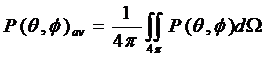So, the directivity,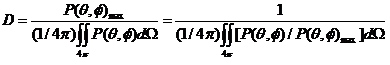OrThe smaller the beam area ΩA, the larger the directivity D. In decibels,The numerical value of D always lies between 1 and ∞. The idealized isotropic antenna radiates equally in all directions, so its beam area ΩA = 4π sr.

Its directivity is,This is the lowest possible directivity (D = 1). All actual antennas have directivities greater than 1 (D > 1).

## Antenna Gain

In electromagnetics, an antenna’s power gain or simply gain is a key performance number that combines the antenna’s directivity and electrical efficiency.

As a transmitting antenna, the gain describes how well the antenna converts input power into radio waves headed in a specified direction.

As a receiving antenna, the gain describes how well the antenna converts radio waves arriving from a specified direction into electrical power.

When no direction is specified, “gain” refers to the peak value of the gain. The radiation pattern is a plot of the gain as a function of direction.

Gain is related to directivity with antenna efficiency factor as: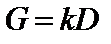k or η is the dimensionless antenna efficiency factor (0 ≤ k ≤ 1). If k or η = 1, i.e. for a lossless antenna. In practice, the gain is always less than the directivity D.

Antenna Gain can be of two types-

1. Power Gain (Gp)
2. Directive Gain (Gd)

Power Gain (Gp): It is the ratio of radiation intensity in a given direction to the average total input power.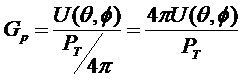Total input power PT = Pr + Pl

Pl: Ohmic losses in the antenna

Directive Gain (Gd): The ratio of radiation intensity in a particular direction to the average radiated power.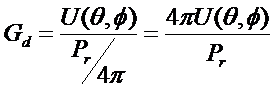Gd does not depend upon the power input to the antenna & its ohmic losses.

The maximum value of directive gain is the directivity D of the antenna.

Also,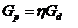η: Efficiency factor which lies between 0 to 1

### What is the Difference Between Antenna Gain and Directivity?

• Directivity defines the concentration of radiated power in a particular direction, whereas Gain specifies the concentration of input power in a particular direction.
• Gain is the combination of directivity and electrical efficiency.
• Gain is always less than the directivity because most of the antennas have some internal losses. Also, the gain is a practical and measurable term.

You can also check this Antenna Calculator to determine the correct antenna down-tilt angle given the heights of the antennas and the distance between them.

The author is a final year B.Tech ECE student at RSET, Cochin

The article was first published on 22 July 2016 and recently updated on 09 December 2022.

1.Nikhil

Thanks

2.Nitin

fundamentals nicely explained

3.Gang Lv

Good, I search the net for the content of attenna gain. But only this one is the best tutorial material and it is very helpful, I think.

•EFY Team

4.Nikz

nice job. but i have few doubts if u could help me with. i want to know if i plug antenna with higher gain to a router, which has antenna with lower gain will it effect to the router in long term?

5.Aman Kamboj

Hi Can you please tell me: which antenna is better from below:
1. Having 30dBi Min gain
2. Having 15dB Min Gain